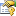﻿ GaussNewton Properties# GaussNewton Properties

The GaussNewton type exposes the following members.Properties
NameDescriptionConvergence
Gets or sets the convergence verification method.
(Inherited from BaseLeastSquaresMethod.)CurrentIteration
Gets the current iteration number.
(Inherited from BaseLeastSquaresMethod.)Deltas
Gets the vector of coefficient updates computed in the last iteration.Function
Gets or sets a parameterized model function mapping input vectors into output values, whose optimum parameters must be found.
(Inherited from BaseLeastSquaresMethod.)Gradient
Gets or sets a function that computes the gradient vector in respect to the function parameters, given a set of input and output values.
(Inherited from BaseLeastSquaresMethod.)HasConverged
Gets whether the algorithm has converged.
(Inherited from BaseLeastSquaresMethod.)Hessian
Gets the approximate Hessian matrix of second derivatives created during the last algorithm iteration.Iterations Obsolete.
(Inherited from BaseLeastSquaresMethod.)Jacobian
Gets the Jacobian matrix of first derivatives computed in the last iteration.MaxIterations
Gets or sets the maximum number of iterations performed by the iterative algorithm. Default is 100.
(Inherited from BaseLeastSquaresMethod.)NumberOfParameters
Gets the number of variables (free parameters) in the optimization problem.
(Inherited from BaseLeastSquaresMethod.)NumberOfVariables Obsolete.
Gets the number of variables (free parameters) in the optimization problem.
(Inherited from BaseLeastSquaresMethod.)ParallelOptions
Gets or sets the parallelization options for this algorithm.
(Inherited from ParallelLearningBase.)Residuals
Gets the vector of residuals computed in the last iteration. The residuals are computed as (y - f(w, x)), in which y are the expected output values, and f is the parameterized model function.Solution
Gets the solution found, the values of the parameters which optimizes the function, in a least squares sense.
(Inherited from BaseLeastSquaresMethod.)StandardErrors
Gets standard error for each parameter in the solution.Token
Gets or sets a cancellation token that can be used to cancel the algorithm while it is running.
(Inherited from ParallelLearningBase.)Tolerance
Gets or sets the maximum relative change in the watched value after an iteration of the algorithm used to detect convergence. Default is zero.
(Inherited from BaseLeastSquaresMethod.)Value
Gets the value at the solution found. This should be the minimum value found for the objective function.
(Inherited from BaseLeastSquaresMethod.)
TopSee Also